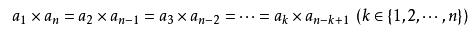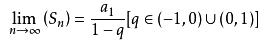# 等比数列和公式介绍

## 等比数列和公式介绍

2017-01-03 作者: xuzhiping 浏览: 7515 次

（2）通项公式（等比数列通项公式经过界说式叠乘而来）

（3）求和公式：

$$S_n=na_1$$（q=1）

$$S_n= \frac { a_1(1-q^n) }{1-q}$$

Sn=首相（1-末项）/1-公比（公比≠1）

（4）从等比数列的界说、通项公式、前n项和公式能够推出：（5）等比中项：若a_qa_p=[a_r]^2，那么a_r为a_p，a_q等比中项。

$$a_{n-1}a_{n+1}=[a_n]^2$$或许$$\frac {a_n} {a_{n-1}} = \frac {a_{n+1}} {a_n}$$。

（6）无量递缩等比数列各项和公式：(7)由等比数列组成的新的等比数列的公比：

{an}是公比为q的等比数列

1.若A=a1+a2+……+an

B=an+1+……+a2n

C=a2n+1+……a3n

2.若A=a1+a4+a7+……+a3n-2

B=a2+a5+a8+……+a3n-1

C=a3+a6+a9+……+a3n